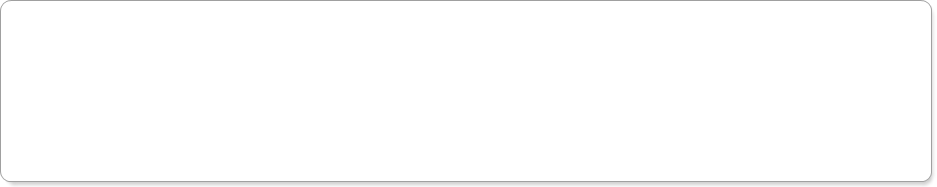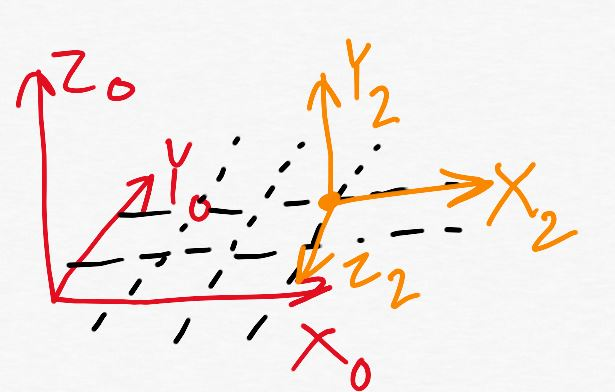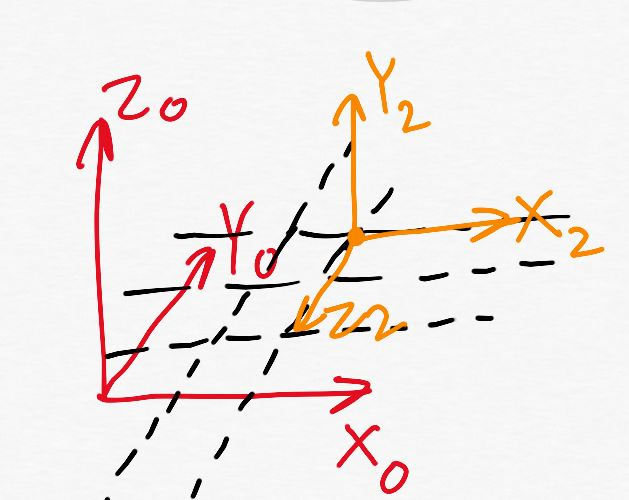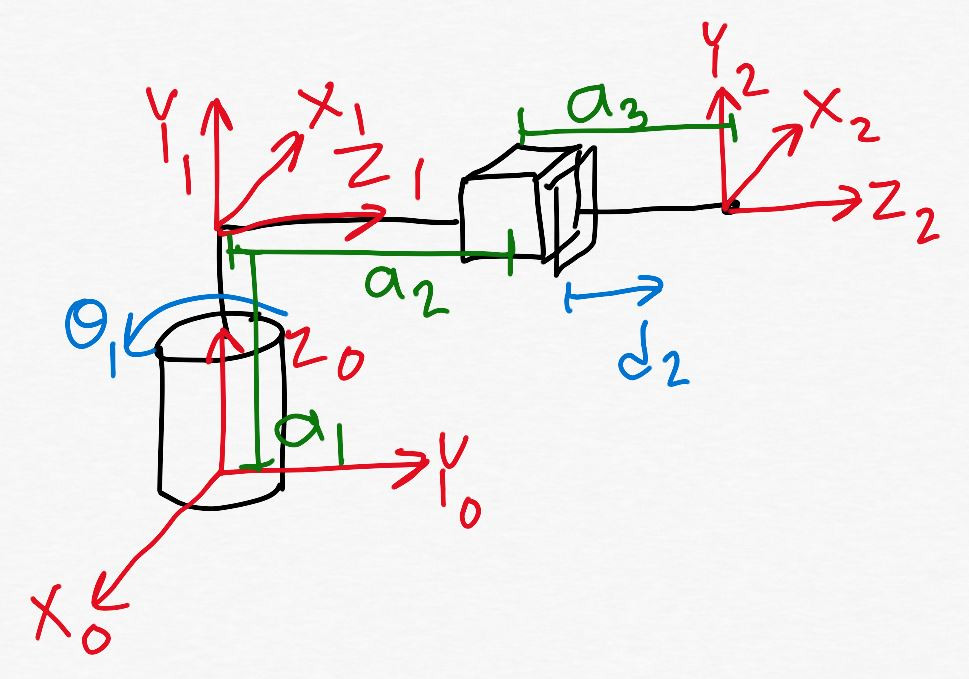Robotics 1
Homogeneous Transformation Matrix
QuizQuestion 2:  In a homogeneous transformation matrix, one of the parts is the displacement vector.  Which of the homogeneous transformation matrices shown here has the displacement vector highlighted in yellow?
Question 4:  Suppose that you have a kinematic diagram with frame 2 on the end-effector, and suppose you have found the two homogeneous transformation matrices as shown here.  What is the X position of the end-effector in the base frame (that is, the 0 frame)?
Question 5:  Shown here is a kinematic diagram.  Which of these is the correct homogeneous transformation matrix from frame 0 to frame 1?
Questions 7-10:  Shown here is a kinematic diagram.  When the joint variables and link lengths are as shown here, what is the homogeneous transformation matrix from the base frame to the end-effector frame?  The given values are correct - fill in the missing values.  Keep in mind that you can use any combination of hand calculations and calculations from your programming to help you solve the problem.  Round your answers to 3 places after the decimal.  (Note: graduate students might want to do problems 11-15 before doing this problem.)
Questions 11-15: Shown here is a kinematic diagram and a Denavit-Hartenberg parameter table.  In each blank space, select from the drop-down list the correct entry.  A few entries are given for you.
Question 1: In a homogeneous transformation matrix, one of the parts is the rotation matrix.  Which of the homogeneous transformation matrices shown here has the rotation matrix highlighted in yellow?None of these is correct.None of these is correct.None of these is correct.
7
4
3
9
Question 3:  Which of these shows how we can combine homogeneous transformation matrices together?None of these is correct.None of these is correct.Question 6: Suppose that I have some manipulator of unknown type that has two degrees of freedom.  With some values of link lengths and joint variables, I found the homogeneous transformation matrix from the base frame to the end-effector frame to be as shown here.  Which picture correctly shows the location and orientation of the end-effector?None of these is correct.0
0
0
1
0
-0.259
1
0
0
1
-0.259
00
0
0